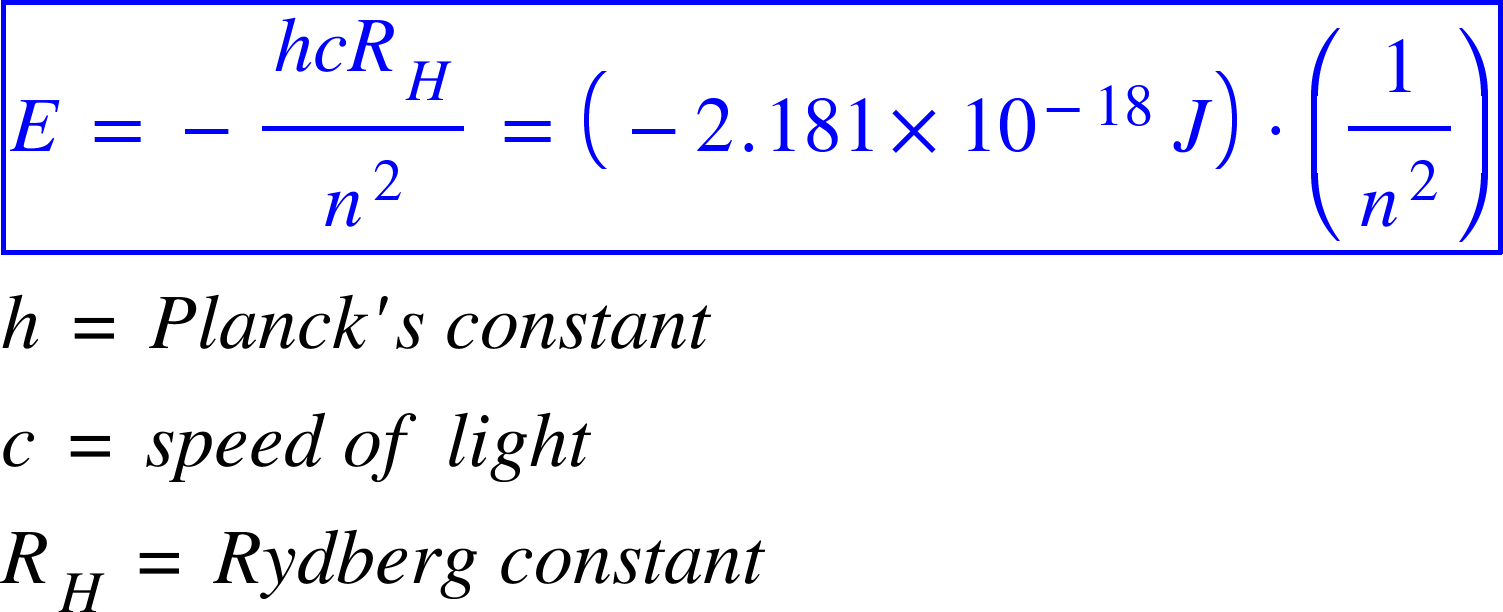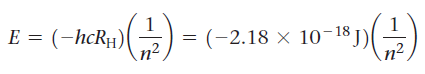# Problem: Using equation, calculate the energy of an electron in the hydrogen atom when n = 6.

###### FREE Expert Solution

The energy of an electron in a hydrogen atom is given by:The energy level or the shell, in which an electron revolves around the nucleus is termed as n.

In this case: We have n=6.

81% (111 ratings)###### Problem Details

Using equationcalculate the energy of an electron in the hydrogen atom when n = 6.